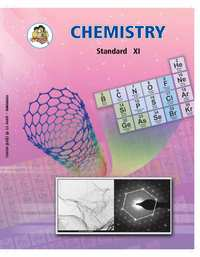# Balbharati solutions for Chemistry 11th Standard Maharashtra State Board chapter 8 - Elements of Group 1 and 2 [Latest edition]

#### Chapters## Chapter 8: Elements of Group 1 and 2

Exercises
Exercises [Page 122]

### Balbharati solutions for Chemistry 11th Standard Maharashtra State Board Chapter 8 Elements of Group 1 and 2 Exercises [Page 122]

Exercises | Q 1. (A) | Page 122

Explain the following.

Hydrogen shows similarity with alkali metals as well as halogens.

Exercises | Q 1. (B) | Page 122

Explain the following.

Standard reduction potential of alkali metals have high negative values.

Exercises | Q 1. (C) | Page 122

Explain the following.

Alkaline earth metals have low values of electronegativity; which decreases down the group.

Exercises | Q 1. (D) | Page 122

Explain the following.

Sodium dissolves in liquid ammonia to form a solution which shows electrical conductivity.

Exercises | Q 1. (E) | Page 122

Explain the following.

BeCl2 is covalent while MgCl2 is ionic.

Exercises | Q 1. (F) | Page 122

Explain the following.

Lithium floats and water while sodium floats and catches fire when put in water.

Exercises | Q 2. (A) | Page 122

Write a balanced chemical equation for the following.

$\ce{CO2}$ is passed into a concentrated solution of NaCl, which is saturated with $\ce{NH3}$.

Exercises | Q 2. (B) | Page 122

Write a balanced chemical equation for the following.

A 50% solution of sulphuric acid is subjected to electrolyte oxidation and the product is hydrolysed.

Exercises | Q 2. (C) | Page 122

Write a balanced chemical equation for the following.

Magnesium is heated in air.

Exercises | Q 2. (D) | Page 122

Write a balanced chemical equation for the following.

Beryllium oxide is treated separately with aqueous HCl and aqueous NaOH solutions.

Exercises | Q 3. (A) | Page 122

Describe the diagonal relationship between Li and Mg with the help of two illustrative properties.

Exercises | Q 3. (B) | Page 122

Outline the industrial production of dihydrogen from steam. Also, write the chemical reaction involved.

Exercises | Q 3. (C) | Page 122

A water sample, which did not give lather with soap, was found to contain $\ce{Ca(HCO3)2}$ and $\ce{Mg(HCO3)2}$. Which chemical will make this water give lather with soap? Explain with the help of chemical reactions.

Exercises | Q 3. (D) | Page 122

Name the isotopes of hydrogen. Write their atomic composition schematically and explain which of these is radioactive?

Exercises | Q 4. (A) | Page 122

Name the following.

Alkali metal with smallest atom.

Exercises | Q 4. (B) | Page 122

Name the following.

The most abundant element in the universe.

Exercises | Q 4. (C) | Page 122

Name the following.

Exercises | Q 4. (D) | Page 122

Name the following.

Ions having high concentration in cell sap.

Exercises | Q 4. (E) | Page 122

Name the following.

A compound having hydrogen, aluminium, and lithium as its constituent elements.

Exercises | Q 5. (A) | Page 122

Choose the correct option.

The unstable isotope of hydrogen is _________

• H-1

• H-2

• H-3

• all the three

Exercises | Q 5. (B) | Page 122

Choose the correct option.

Identify the odd one.

• Rb

• Ra

• Sr

• Be

Exercises | Q 5. (C) | Page 122

Choose the correct option.

Which of the following is Lewis acid?

• BaCl2

• KCl

• BeCl2

• LiCl

Exercises | Q 5. (D) | Page 122

Choose the correct option.

What happens when crystalline Na2CO3 is heated?

• releases CO2

• loses H2O

• decomposes in NaHCO3

• no change taken place

## Chapter 8: Elements of Group 1 and 2

Exercises## Balbharati solutions for Chemistry 11th Standard Maharashtra State Board chapter 8 - Elements of Group 1 and 2

Balbharati solutions for Chemistry 11th Standard Maharashtra State Board chapter 8 (Elements of Group 1 and 2) include all questions with solution and detail explanation. This will clear students doubts about any question and improve application skills while preparing for board exams. The detailed, step-by-step solutions will help you understand the concepts better and clear your confusions, if any. Shaalaa.com has the Maharashtra State Board Chemistry 11th Standard Maharashtra State Board solutions in a manner that help students grasp basic concepts better and faster.

Further, we at Shaalaa.com provide such solutions so that students can prepare for written exams. Balbharati textbook solutions can be a core help for self-study and acts as a perfect self-help guidance for students.

Concepts covered in Chemistry 11th Standard Maharashtra State Board chapter 8 Elements of Group 1 and 2 are Hydrogen, Alkali Metals and Alkaline Earth Metals, Some Important Compounds of Elements of S-block.

Using Balbharati 11th solutions Elements of Group 1 and 2 exercise by students are an easy way to prepare for the exams, as they involve solutions arranged chapter-wise also page wise. The questions involved in Balbharati Solutions are important questions that can be asked in the final exam. Maximum students of Maharashtra State Board 11th prefer Balbharati Textbook Solutions to score more in exam.

Get the free view of chapter 8 Elements of Group 1 and 2 11th extra questions for Chemistry 11th Standard Maharashtra State Board and can use Shaalaa.com to keep it handy for your exam preparation